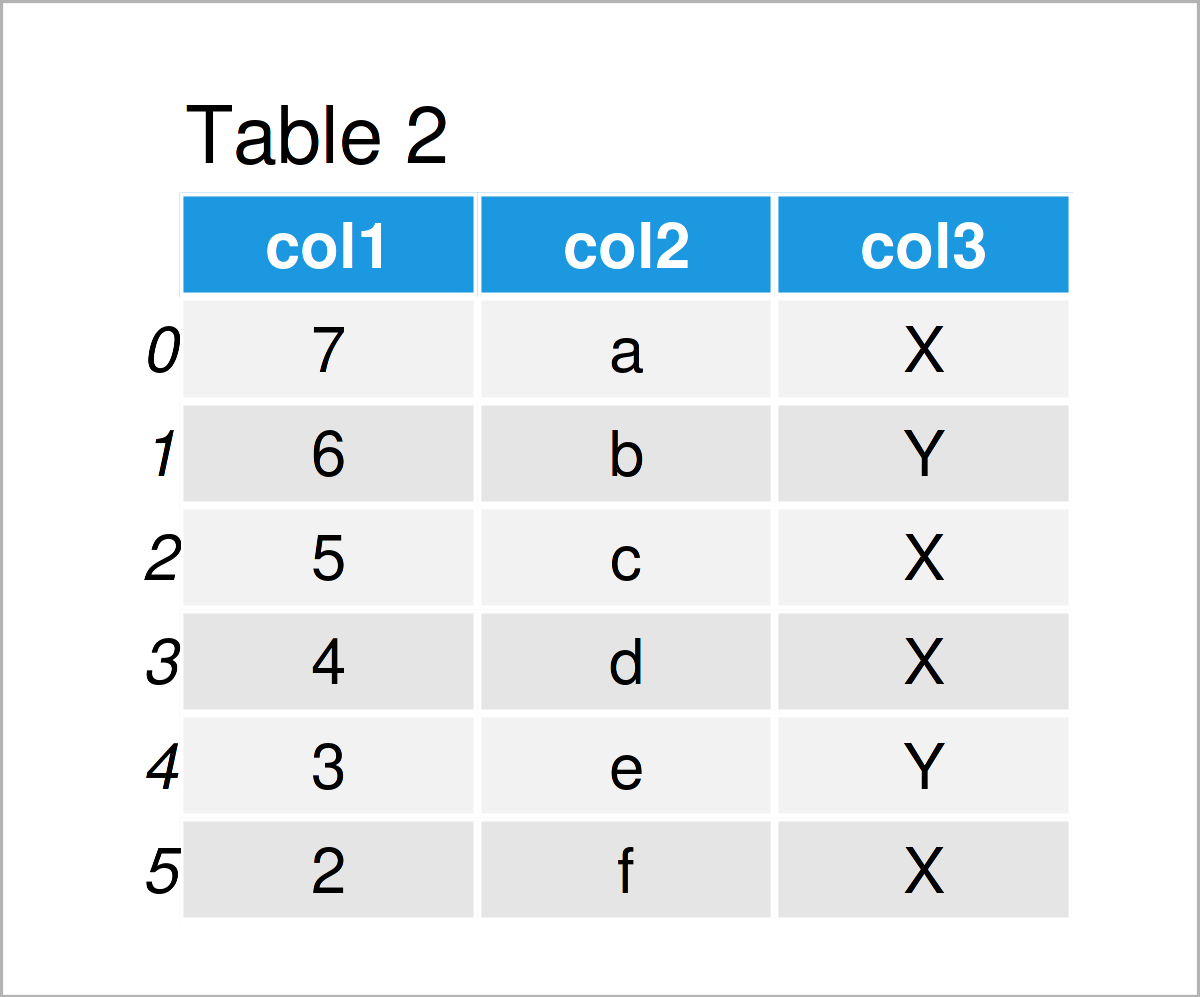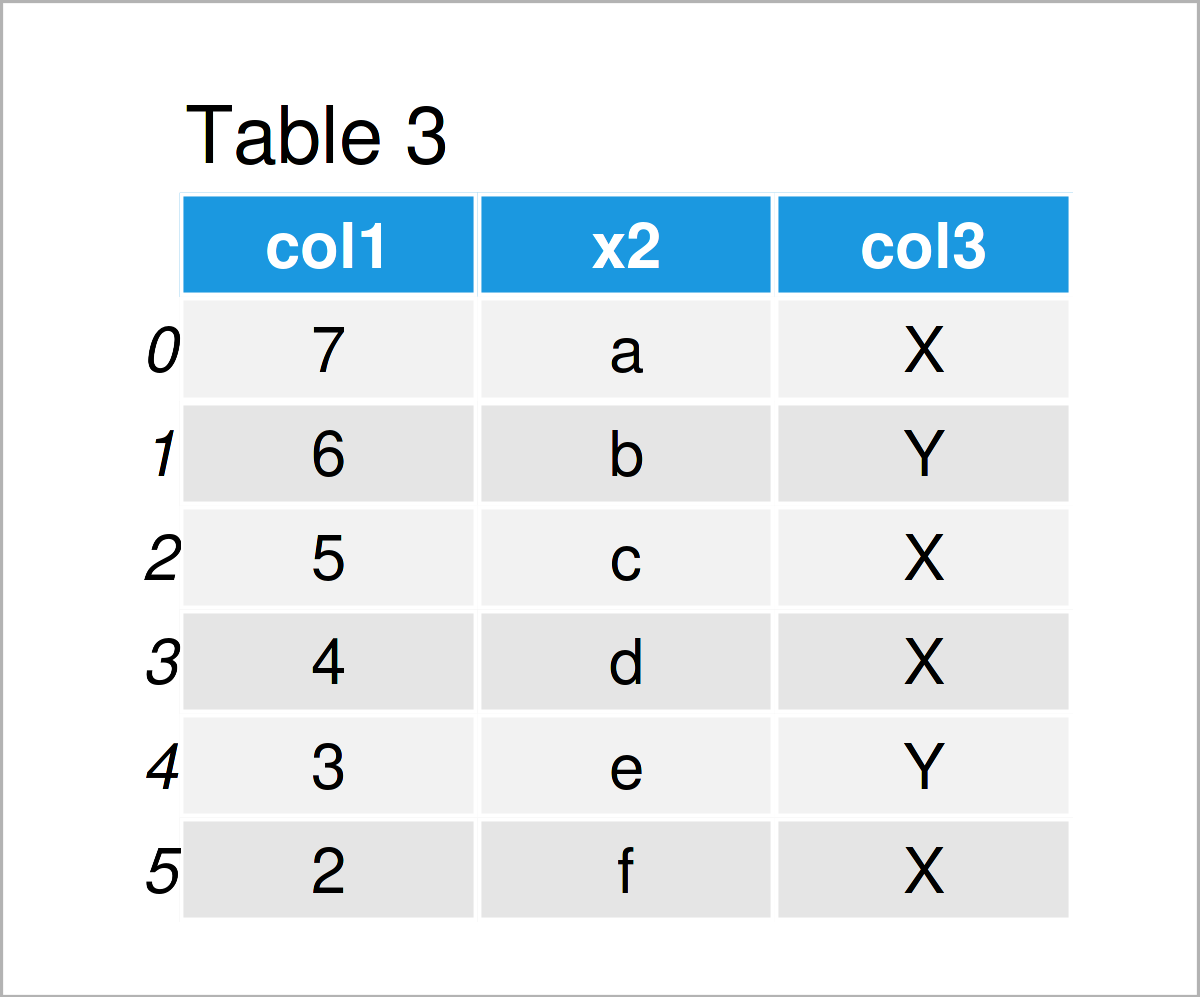# Rename Columns of pandas DataFrame in Python (2 Examples)

In this Python tutorial you’ll learn how to modify the names of columns in a pandas DataFrame.

The tutorial consists of two examples for the modification of the column names in a pandas DataFrame. To be more specific, the article will contain this information:

Let’s dive into it.

## Example Data & Add-On Packages

To be able to use the functions of the pandas library, we first need to import pandas:

`import pandas as pd                                         # Load pandas`

Furthermore, consider the following example data:

```data = pd.DataFrame({"x1":range(7, 1, - 1),                 # Create pandas DataFrame
"x2":["a", "b", "c", "d", "e", "f"],
"x3":["X", "Y", "X", "X", "Y", "X"]})
print(data)                                                 # Print pandas DataFrame```As you can see based on Table 1, our example data is a DataFrame composed of six rows and three columns. The variables in our DataFrame are called x1, x2, and x3.

## Example 1: Change Names of All Variables Using columns Attribute

Example 1 explains how to rename the column names of all variables in a data set.

The following Python code uses the columns attribute to create a copy of our DataFrame where the original header is replaced by the new column names col1, col2, and col3.

```data_new1 = data.copy()                                     # Create copy of DataFrame
data_new1.columns = ["col1", "col2", "col3"]                # Using columns attribute
print(data_new1)                                            # Print updated pandas DataFrame```In Table 2 you can see that we have created a new pandas DataFrame with updated variables names by running the previous Python programming code.

## Example 2: Change Names of Specific Variables Using rename() Function

The Python programming code below shows how to exchange only some particular column names in a pandas DataFrame.

For this, we can use the rename function as shown below:

```data_new2 = data.copy()                                     # Create copy of DataFrame
data_new2 = data_new2.rename(columns = {"x1": "col1", "x3": "col3"})  # Using rename()
print(data_new2)                                            # Print updated pandas DataFrame```As shown in Table 3, we have created another duplicate of our input data matrix, in which we have only renamed the columns x1 and x3.

## Video & Further Resources on this Topic

In case you need further info on the examples of this tutorial, I recommend having a look at the following video on my YouTube channel. In the video instruction, I’m explaining the Python programming syntax of this article in more detail.

Do you need further information on the contents of this article? Then you could have a look at the following video on the Absent Data YouTube channel. In the video, the speaker illustrates the contents of this tutorial in a live session in Python.

In addition, you might read the related Python programming articles on this website:

To summarize: At this point you should know how to rename the names of variables in a pandas DataFrame in the Python programming language. If you have further questions, please tell me about it in the comments.

Subscribe to the Statistics Globe Newsletter